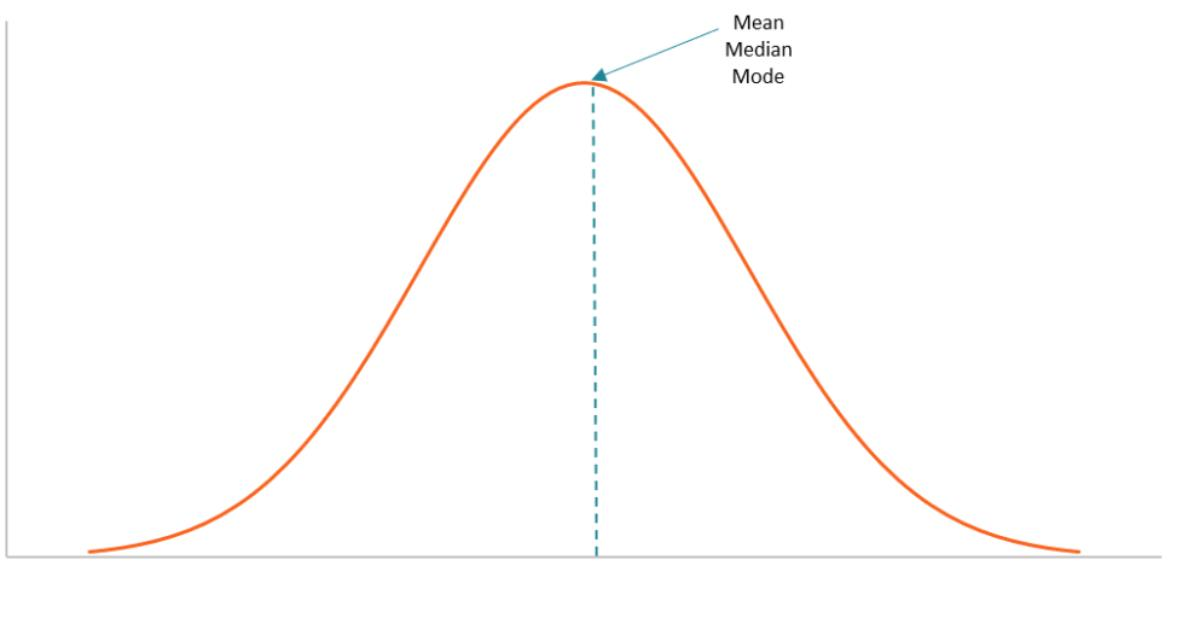### Magazine# Central Tendency

last update Friday, February 21, 2020

tags

#  #  # #

The term central tendency refers to the "middle" value or perhaps a typical value of the data and is measured using the mean, median, or mode. Each of these measures is calculated differently, and the one that is best to use depends upon the situation.

### Mean

The mean is the most commonly-used measure of central tendency. When we talk about an "average", we usually are referring to the mean. The mean is simply the sum of the values divided by the total number of items in the set. The result is referred to as the arithmetic mean. Sometimes it is useful to give more weighting to certain data points, in which case the result is called the weighted arithmetic mean.

The notation used to express the mean depends on whether we are talking about the population mean or the sample mean:

μ =  population mean

× =  sample mean

The population means then is defined as:

 1 - N
N
 xi
μ =
i=1

where

N =  number of data points in the population

xi =  value of each data point i.

The mean is valid only for interval data or ratio data. Since it uses the values of all of the data points in the population or sample, the mean is influenced by outliers that may be at the extremes of the data set.

### Median

The median is determined by sorting the data set from lowest to highest values and taking the data point in the middle of the sequence. There is an equal number of points above and below the median.
For example, in the data set {1,2,3,4,5} the median is 3; there are two data points greater than this value and two data points less than this value. In this case, the median is equal to the mean.
But consider the data set {1,2,3,4,10}. In this dataset, the median still is three, but the mean is equal to 4. If there is an even number of data points in the set, then there is no single point at the middle and the median is calculated by taking the mean of the two middle points.

The median can be determined for ordinal data as well as interval and ratio data. Unlike the mean, the median is not influenced by outliers at the extremes of the data set. For this reason, the median often is used when there are a few extreme values that could greatly influence the mean and distort what might be considered typical. This often is the case with home prices and with income data for a group of people, which often is very skewed. For such data, the median often is reported instead of the mean. For example, in a group of people, if the salary of one person is 10 times the mean, the mean salary of the group will be higher because of the unusually large salary. In this case, the median may better represent the typical salary level of the group.

### Mode

The mode is the most frequently occurring value in the data set. For example, in the data set {1,2,3,4,4}, the mode is equal to 4. A data set can have more than a single mode, in which case it is multimodal. In the data set {1,1,2,3,3} there are two modes: 1 and 3.

The mode can be very useful for dealing with categorical data. For example, if a sandwich shop sells 10 different types of sandwiches, the mode would represent the most popular sandwich. The mode also can be used with ordinal, interval, and ratio data. However, in interval and ratio scales, the data may be spread thinly with no data points having the same value. In such cases, the mode may not exist or may not be very meaningful.

### When to use Mean, Median, and Mode

The following table summarizes the appropriate methods of determining the middle or typical value of a data set based on the measurement scale of the data.

 Measurement Scale Best Measure of the "Middle" Nominal(Categorical) Mode Ordinal Median Interval Symmetrical data: MeanSkewed data: Median Ratio Symmetrical data: MeanSkewed data: Median

The End, what is next?

Note: the page content was squeezed for you with a focus on , by Paperfree Magazine Team, editor -

check this out, Central Tendency

Want more content related to "" ? Subscribe PaperFree  Magazine!

We will send an email from PaperFree Magazine with the top content on this subject: , .